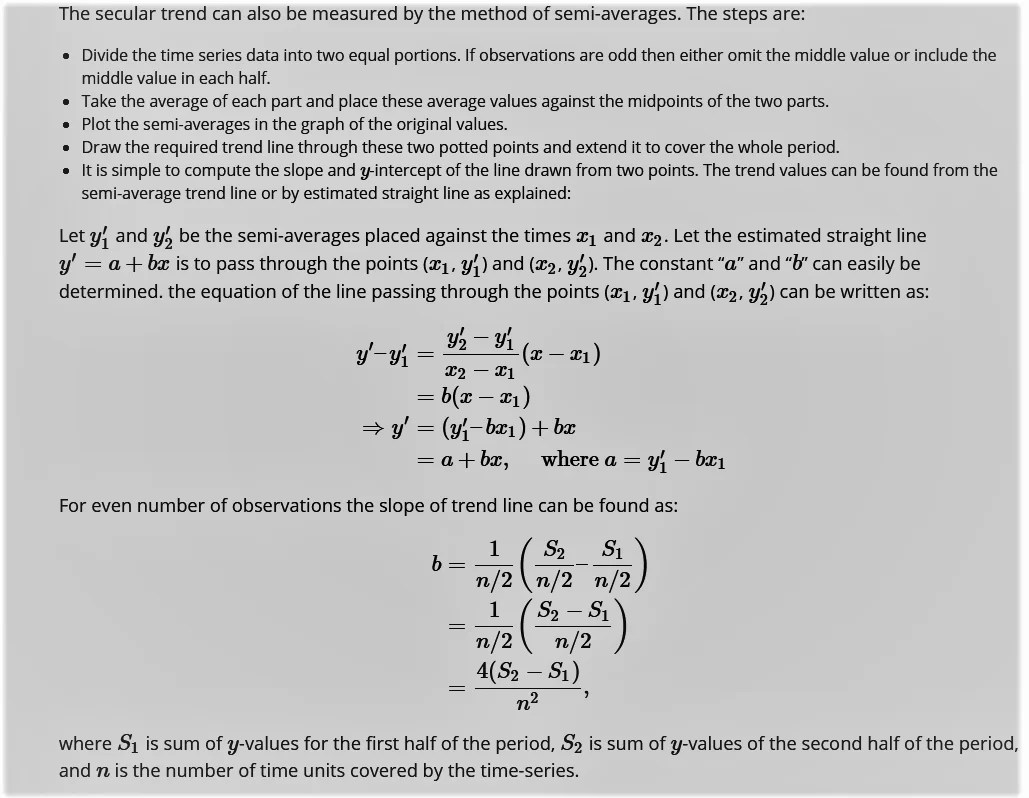# Semi Average Method

4th May 2021

Under this method, as the name itself suggests semiaverages are calculated to find out the trend values. By semi-averages is meant the averages of the two halves of a series. In this method, thus, the given series is divided into two equal parts (halves) and the arithmetic mean of the values of each part (half) is calculated. The computed means are termed as semi-averages. Each semi-average is paired with the centre of time period of its part. The two pairs are then plotted on a graph paper and the points are joined by a straight line to get the trend. It should be noted that if the data is for even number of years, it can be easily divided into two halves. But if it is for odd number of years, we leave the middle year of the time series and two halves constitute the periods on each side of the middle year.